### Journal of Advances in Nanotechnology

Current Issue Volume No: 1 Issue No: 1

ISSN: 2689-2855

Research Article Open Access
• Available online freely Peer Reviewed
• ### Marangoni Effect in Second Grade Forced Convective Flow of Water Based Nanofluid

Ghulam Rasool 1   Ting Zhang 1   Anum Shafiq 2

1School of Mathematical Sciences, Zhejiang University, Hangzhou 310027, PR-China

2School of Mathematics and Statistics, Nanjing University of Information Science and Technology, Nanjing 210044, China

## Abstract

Advances in nanotechnology especially in nanofluids comprising of a typical base fluid saturated with nano-size metallic particles with enhanced thermophysical properties is one of the hot topics in industrial as well as engineering applications of applied mathematics. This article explores the impact of Lorentz forces and Maragoni effect on second grade nanofluid forced convective flow. A two phase model is chosen to validate the nanofluid. Series solutions are achieved through HAM after transformation of PDEs into ODEs. The Brownian motion effect, Thermophoresis and the Marangoni effect are the main influencing factors for present flow model. In addition, the influence of pertinent fluid parameters such as Schmidt number, Magnetic number and Prandtl number on the velocity, temperature and concentration profiles is discussed with the help of graphs. With an enhanced Marangoni factor the hydraulic boundary layer thickness shows enhancement.

##### Author Contributions
Received 18 Mar 2019; Accepted 28 Mar 2019; Published 03 Apr 2019;

Checked for plagiarism: Yes

Review by: Single-blindThis is an open-access article distributed under the terms of the Creative Commons Attribution License, which permits unrestricted use, distribution, and reproduction in any medium, provided the original author and source are credited.

Competing interests

The authors have declared that no competing interests exist.

Citation:

Ghulam Rasool, Ting Zhang, Anum Shafiq (2019) Marangoni Effect in Second Grade Forced Convective Flow of Water Based Nanofluid. Journal of Advances in Nanotechnology - 1(1):50-61.

## Introduction

The concept of nanofluid is very simple but it has broadly improved the efficiency of fluids especially from industrial point of view. The suspension of nanoparticles in typical base fluid results in a very highly conductive fluid that certainly reduces the human effort as well as the machine heating. These suspensions can meet the cooling requirement for any type of thermal system. The foundation of nanofluid was laid by Choi 1 and later on, the concept became famous in all the related fields of fluid dynamics due to its industrial applications. Ibanez et al. 2 discussed an analytic investigation for MHD nanoliquid flow with suction/injection and radiation effects. Gorji et al. 3 involved a variable magnetic field for investigation of a squeezed nanofluid flow considering the Brinkman model. Related articles can be seen in 4, 5, 6, 7, 8, 9, 10, 11 and references cited therein.

Surface tension as well as the gradients of temperature and concentration results in Marangoni convection appearing in fluid flow analysis. The topic is interesting due to its applications in crystal growth mechanism, beam melting and welding etc. Lin et al. 12 reported the Marangoni convection in nanoliquid flow via thermal gradient varying the magnetic effect. Surface tension is taken as a nonlinear temperature and concentration function. Aly and Ebaid 13 found exact solutions considering Marangoni effect in nanofluid flow using Laplace transformation method. Most recent articles can be seen in 14, 15, 16, 17 and reference cited therein.

Present inspiration is covered by the following novel aspects. Firstly, to model a second grade nanofluid forced convective flow due to the Lorentz forces instigated into the fluid by induction of a variable magnetic field. Secondly, to achieve series solutions through HAM. The PDEs are transformed into ODEs using usual similarity transformations. Finally, to discuss the results through graphs with sufficient concluding remarks.

## Problem Statement

We consider a second grade nanofluid forced convection due to the Lorentz forces instigated into the model by induction of an applied Magnetic field. The effect of magnetic field develops normal to the surface upon which the fluid is flowing. The Marangoni effect is utilized to apprehend the fluid flow in forward direction. The problem is considered in two dimensions such that the fluid flows along x-axis and the y-axis extends normal to the surface. There is no fluid motion along y-axis therefore, v=0 is taken. Along x-axis the fluid undergoes the Lorentz forces that are induced into the model due to the applied magnetic field neglecting the Hall effects. The interface temperature is taken as function of x as depicted in Figure 1. The interface condition and velocity components can be visualized in Figure 1.

Figure 1. Schematic diagram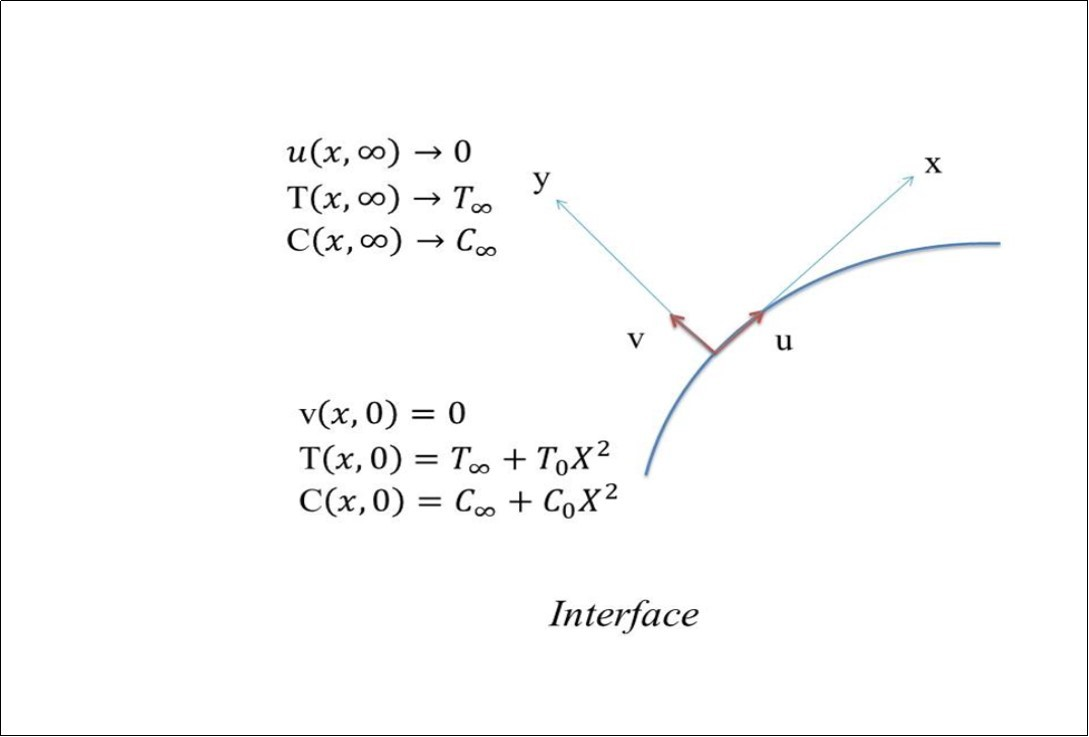Governing equations are therefore, as follows: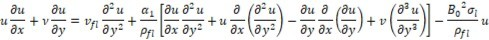……. (2)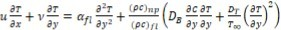……. (3)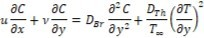……. (4)

with following boundary conditions,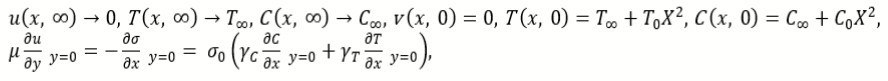….. (5)

The surface tension σ, being a function of T and C can be defined as follows: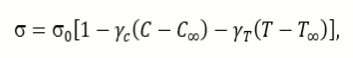……… (6)

where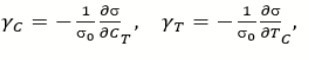………. (7)

Here u , ν are the horizontal and vertical velocity components, respectively v fl. is the kinematic viscosity, ρfl is the density of fluid B0 is the magnetic effect involved in the model for MHD, σ is the surface tension, αfl is is thermal diffusivity of the fluid, the ratio between heat capacity of the nanoparticles (ρc)np and heat capacity of base fluid (ρc) fl. DBr is Brownian diffusion, DTh is Thermophoresis.

Define,

……… (8)

Using (8) in equations 1, 2, 3, 4, we get

…………. (9)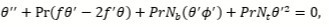……… (10)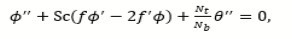………….. (11)

subject to the following boundary conditions: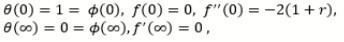……… (12)

where r=(C0 𝜸C)/(T0 γT ), M=(L2 σl B0)/μ, Pr=νflfl is the Prandtl number Nb=(ρc)np DB𝜸 C0 x2) / (ρc)lf L2 a), Nt=(ρc)np DTh x2) / (ρc)lf L2 a), L= μν fl)/(σ0 T0 γT) are the Marangoni factor (r), modified Hartman number (M), Prandtl factor (Pr), Brownian motion parameter (Nb), Schmidt number (Sc), Thermophoresis parameter (Nt) and reference length (L), respectively. The second grade fluid parameter (α) is defined as α1/(ρfl L2).

## Series Solutions

Homotopy analysis method 18, 19, 20, 21, 22 for convergent series solutions is very convenient to obtain the approximated solutions for a given nonlinear system. The method is independent of small/large physical parameters. Thus it is an efficient method as compared to other conventional methods for solving nonlinear systems. Assuming the following initial guesses,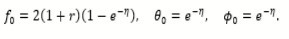…. (13)

The auxiliary parameters can be defined as follows: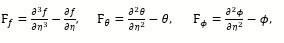……. (14)

such that,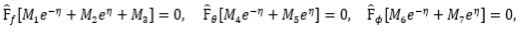……. (15)

where Mi are constants for i=1-7. Subsequently, the zeroth order problems of deformation are written as follows:

with following boundary conditions:

Therefore,

where p (0,1) is a typical embedding parameter and h ̂f , h ̂θ, h ̂ϕ are so-called auxiliary parameters with Nf, Nθ, Nϕ are the non-linear operators. For p =0,1 , we have: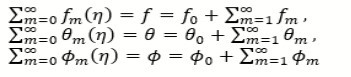……. (19)

The mth problems of deformation are

where gm=1 for m>1 otherwise 0.

Finally,

Thus,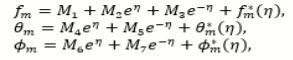…… (22)

are the general solutions where Mi are the arbitrary constants for i=1-7 and fm* (η) ,θm* (η), ϕm* (η) are special solutions.

## Convergence Analysis

The auxiliary parameters introduced in (series solution) for the velocity profile (f), temperature distribution (θ) and concentration distribution (ϕ) are termed as convergence control parameters. These parameters are significant to speed-up the convergence. Convergence intervals are sketched in Figure 2. The interval of interest for convergence of the aforementioned profiles is (-0.60, 0.30).

Figure 2. H-Curves for convergent series solutions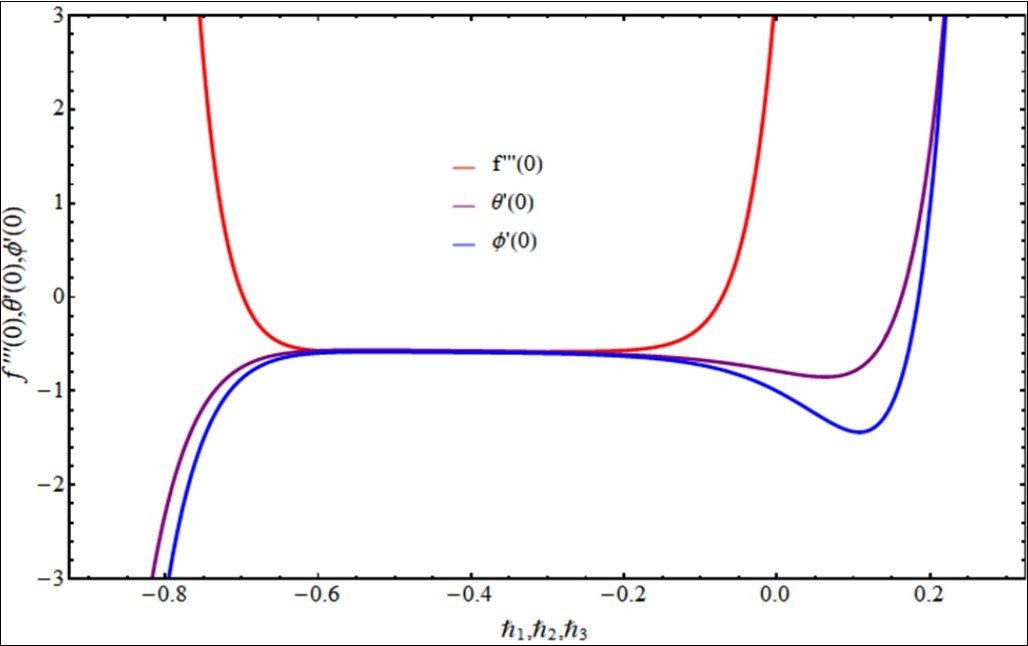## Results and Discussion

This section is concerned with the discussion on graphical results that are obtained through Mathematica based HAM code for specific values of pertinent fluid parameters including the second grade fluid parameter, the Magnetic number, the Marangoni ratio, the Prandtl and Schmidt numbers as well as the Brownian and Thermophoretic parameters. Figure 3. displays the effect of second grade fluid parameter on the velocity profile. The velocity shows decreasing behavior for incremental values of respective parameter. A certain increase in viscosity results in decrease of fluid motion. The velocity shows reduction with augmented values of Marangoni ratio as depicted in Figure 4. Similar is the case noticed for Magnetic number as displayed in Figure 5. A significant drop in velocity profile appears at first sight however, the variation slows down with the stronger effect of magnetic number. The temperature profile, as displayed in Figure 6, shows augmented behavior with augmented values of Marangoni ratio. However, after certain limitation, the effect can be seen in opposite nature. The Prandtl number shows enhancement in temperature profile due to an enhanced thermal diffiusivity as one can see in Figure 7. Figure 8 and Figure 9 are the display of variation in Temperature profile varying the values of Brownian motion parameter and Thermophoretic parameter, respectively. The rapid and in-predictive movement of nanoparticles as well as the more stronger Thermophoretic force results in incremental behavior of temperature profile. The effect of Marangoni ratio on concentration profile is displayed in Figure 10. The results persist with those appearing in Temperature profile for the same parameter. An opposite behavior is seen upon variation of Thermophoretic parameter and Schmidt number for concentration profile as displayed in Figure 11 and Figure 12, respectively.

Figure 3. Impact of second grade parameter on velocity profile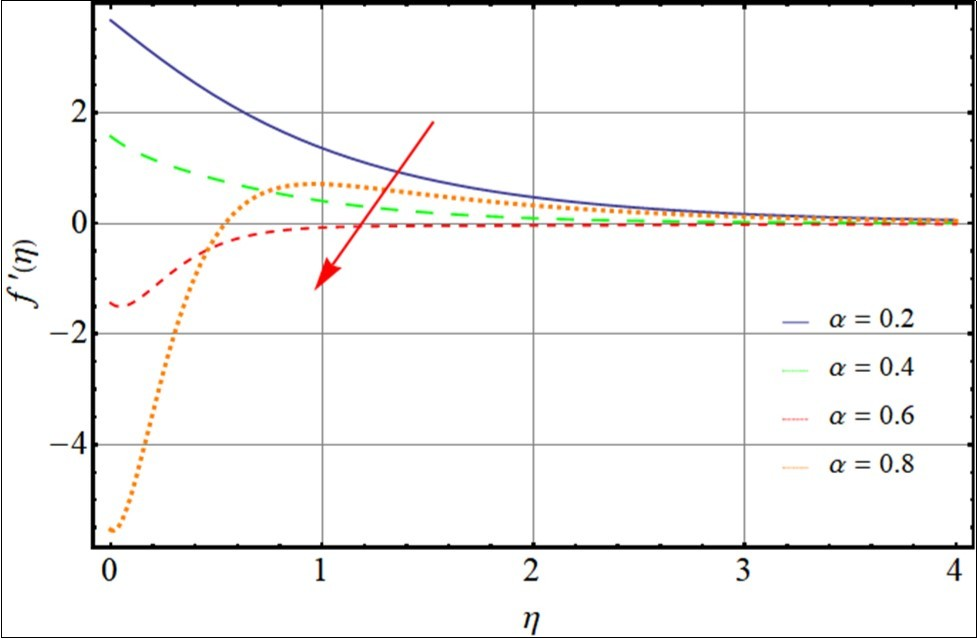Figure 4. Impact of Marangoni ratio on velocity profile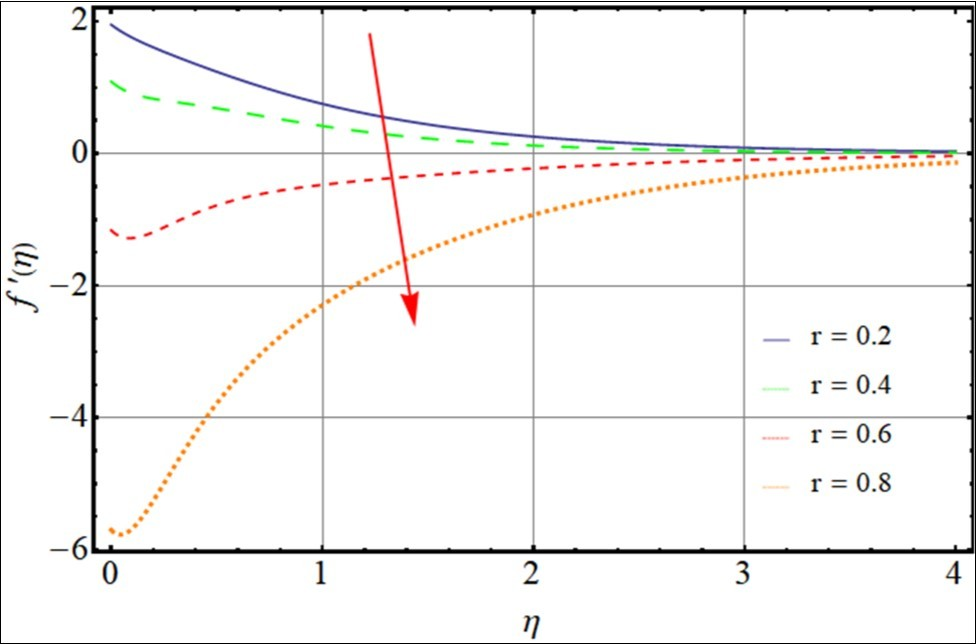Figure 5. Impact of magnetic number on velocity profile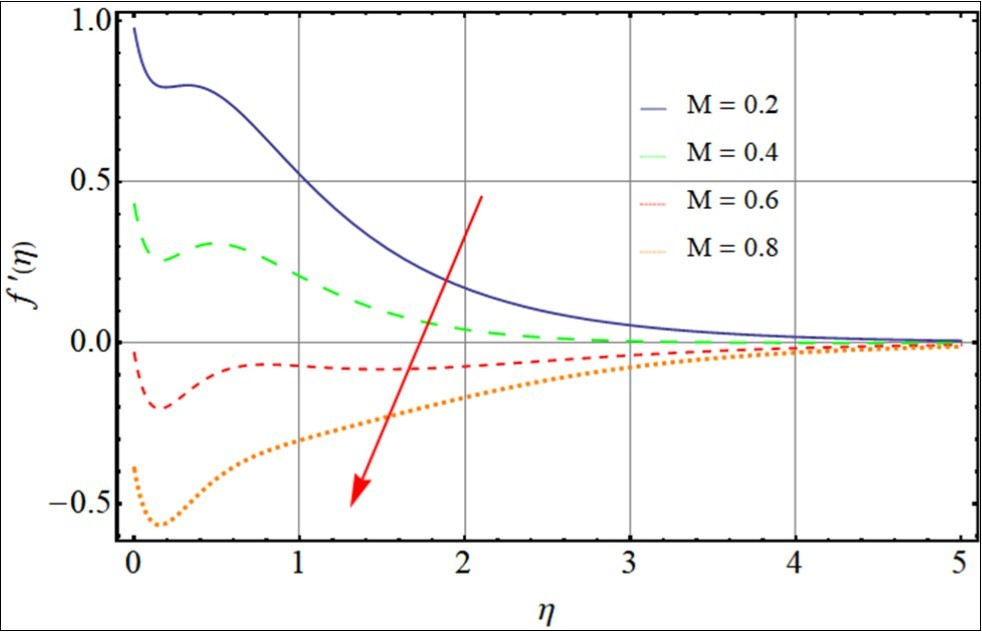Figure 6. Impact of Marangoni ratio on temperature profile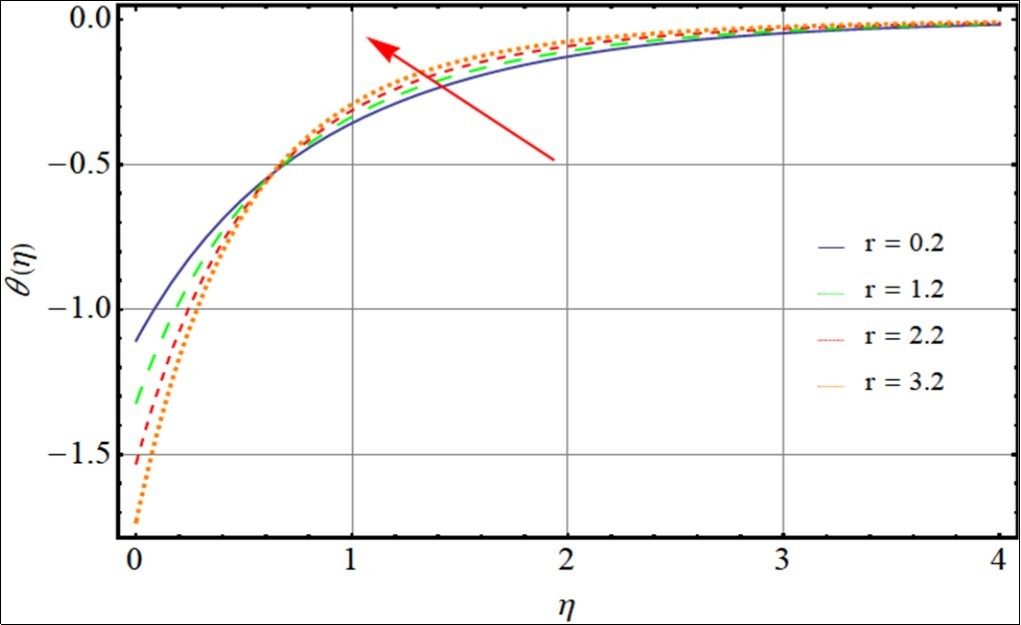Figure 7. Impact of Prandtl number on temperature profile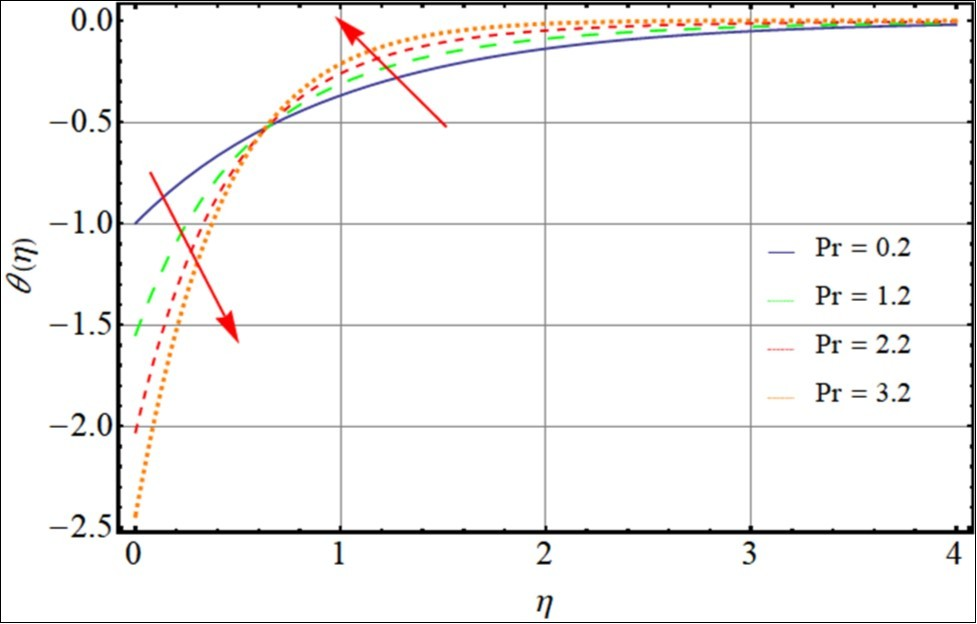Figure 8. Impact of Brownian motion on temperature profile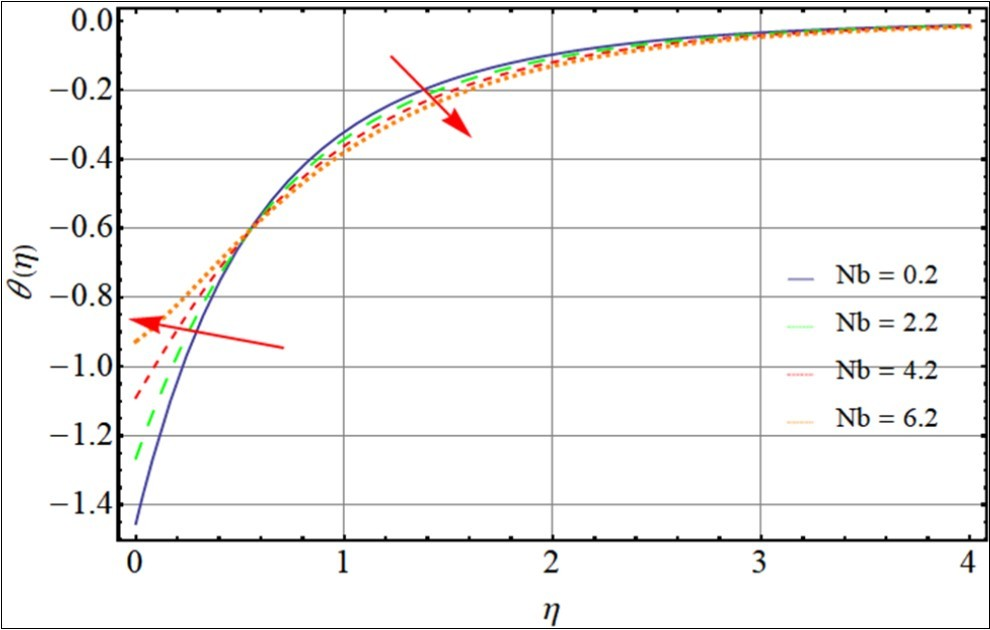Figure 9. Impact of Thermophoresis on temperature profile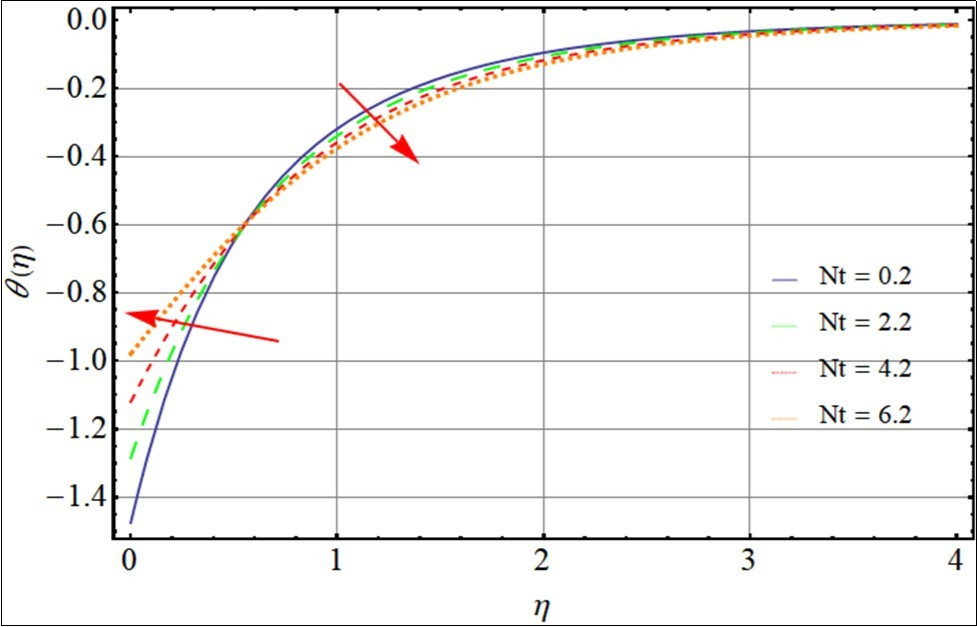Figure 10. Impact of Marangoni ratio on concentration profile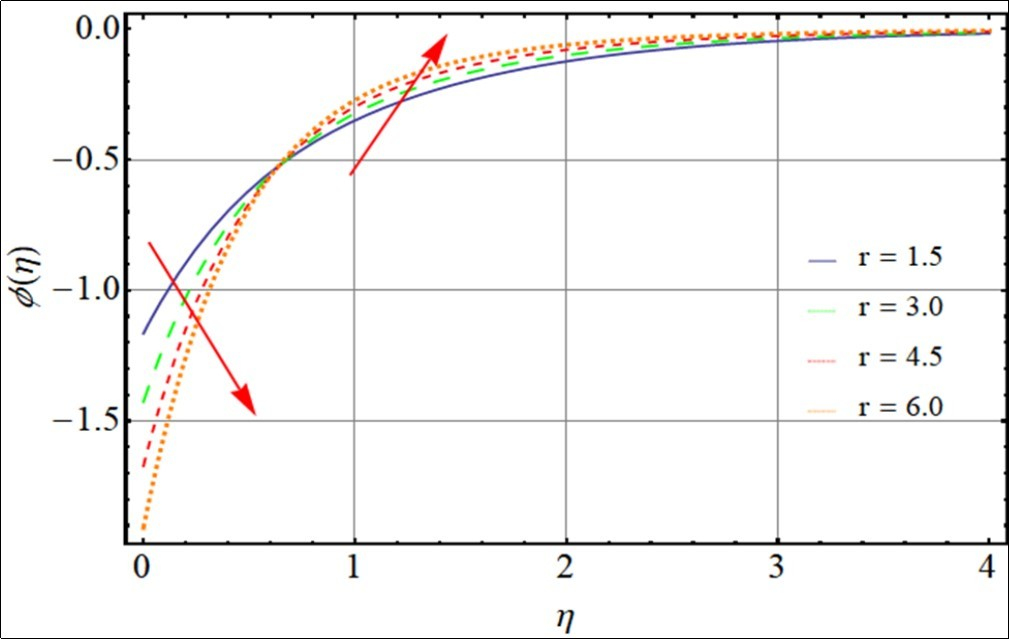Figure 11. Impact of Thermophoresis on concentration profile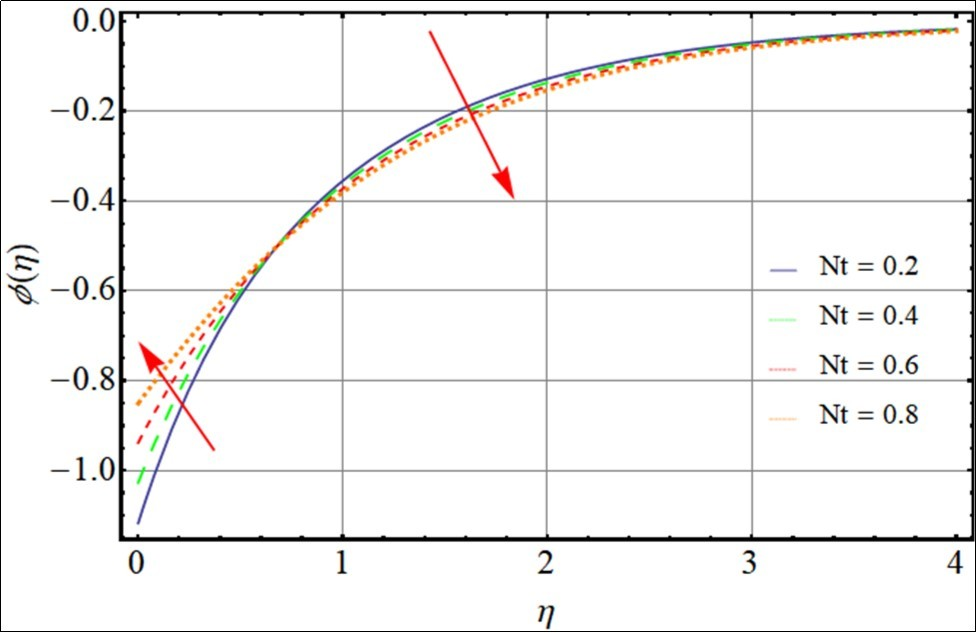Figure 12. Impact of Schmidt number on concentration profile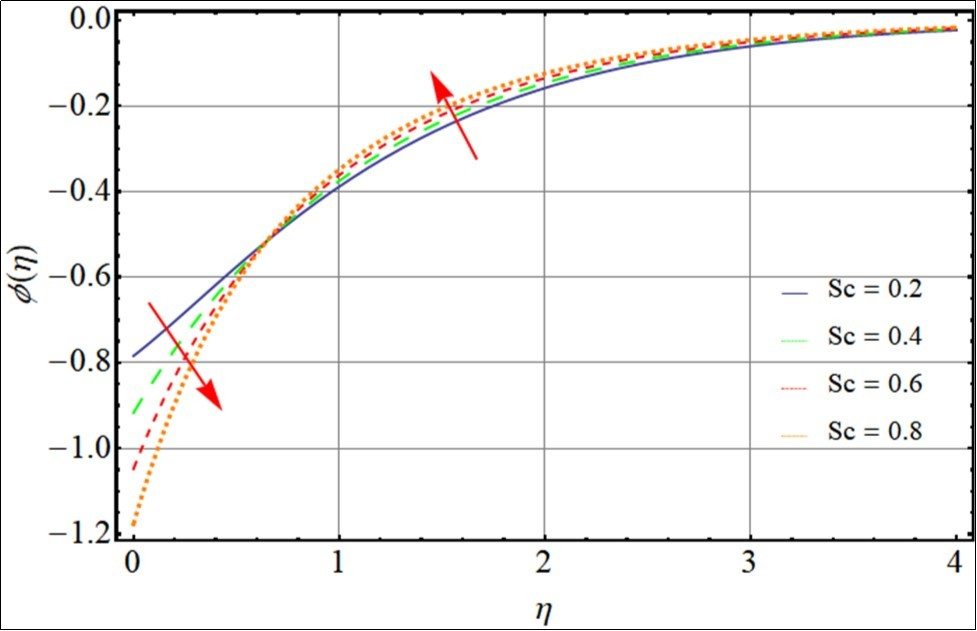## Closing Remarks

This study concludes with the impact of Marangoni effect and Lorentz force generated by MHD on second grade nanofluid flow. Flow model is formulated mathematically in PDEs which are transformed into ODEs using transformation and HAM is applied to get the convergent series solutions. We conclude that velocity profile reduces for stronger Marangoni effect in second grade nanofluid. The Brownian motion and Thermophoresis have significant impact on the flow profiles. Furthermore, the temperature and concentration profile shows augmented behavior with augmented Marangoni ratio. One can see that due to strong impact of Lorentz forces, a reduction in hydraulic boundary layer is noticed however, an opposite behavior is shown the other two profiles.

## Cited by (17)

1.Rasool Ghulam, Shafiq Anum, 2020, Numerical exploration of the features of thermally enhanced chemically reactive radiative Powell–Eyring nanofluid flow via Darcy medium over non-linearly stretching surface affected by a transverse magnetic field and convective boundary conditions, Applied Nanoscience, (), 10.1007/s13204-020-01625-2
2.Ray Atul Kumar, B. Vasu, 2019, Influence of chemically radiative nanoparticles on flow of Maxwell electrically conducting fluid over a convectively heated exponential stretching sheet, World Journal of Engineering, 16(6), 791, 10.1108/WJE-04-2019-0100
3.Khan Sohail A, Hayat T, Alsaedi Ahmed, Zai Q M Zaigham, 2020, Irreversibility analysis in Marangoni forced convection flow of second grade fluid, Journal of Physics Communications, 4(8), 085013, 10.1088/2399-6528/aba8db
4.Shafiq Anum, Khan Ilyas, Rasool Ghulam, Seikh Asiful, Sherif El-Sayed, 2019, Significance of Double Stratification in Stagnation Point Flow of Third-Grade Fluid towards a Radiative Stretching Cylinder, Mathematics, 7(11), 1103, 10.3390/math7111103
5.Sadiq Muhammad Adil, Hayat Tasawar, 2020, Characterization of Marangoni Forced Convection in Casson Nanoliquid Flow with Joule Heating and Irreversibility, Entropy, 22(4), 433, 10.3390/e22040433
6.Rasool Ghulam, Zhang Ting, Chamkha Ali J., Shafiq Anum, Tlili Iskander, et al, 2019, Entropy Generation and Consequences of Binary Chemical Reaction on MHD Darcy–Forchheimer Williamson Nanofluid Flow Over Non-Linearly Stretching Surface, Entropy, 22(1), 18, 10.3390/e22010018
7.Lund Liaquat Ali, Omar Zurni, Khan Ilyas, Sherif El-Sayed M., 2020, Dual Solutions and Stability Analysis of a Hybrid Nanofluid over a Stretching/Shrinking Sheet Executing MHD Flow, Symmetry, 12(2), 276, 10.3390/sym12020276
8.Shafiq Anum, Zari Islam, Rasool Ghulam, Tlili Iskander, Khan Tahir Saeed, 2019, On the MHD Casson Axisymmetric Marangoni Forced Convective Flow of Nanofluids, Mathematics, 7(11), 1087, 10.3390/math7111087
9.Awan Aziz Ullah, Riaz Samia, Sattar Samina, Abro Kashif Ali, 2020, Fractional modeling and synchronization of ferrofluid on free convection flow with magnetolysis, The European Physical Journal Plus, 135(10), 10.1140/epjp/s13360-020-00852-4
10.Rasool Ghulam, Zhang Ting, Shafiq Anum, 2019, Second grade nanofluidic flow past a convectively heated vertical Riga plate, Physica Scripta, 94(12), 125212, 10.1088/1402-4896/ab3990
11.Shafiq Anum, Khan Ilyas, Rasool Ghulam, Sherif El-Sayed M., Sheikh Asiful H., 2020, Influence of Single- and Multi-Wall Carbon Nanotubes on Magnetohydrodynamic Stagnation Point Nanofluid Flow over Variable Thicker Surface with Concave and Convex Effects, Mathematics, 8(1), 104, 10.3390/math8010104
12.Ansari Md Sharifuddin, Magagula Vusi Mpendulo, Trivedi Mumukshu, 2020, Jeffrey nanofluid flow near a Riga plate: Spectral quasilinearization approach, Heat Transfer, 49(3), 1491, 10.1002/htj.21673
13.Hayat T., Khan Sohail A., Alsaedi Ahmed, Fardoun Habib M., 2021, Marangoni Forced Convective Flow of Second Grade Fluid with Irreversibility Analysis and Chemical Reaction, International Journal of Thermophysics, 42(1), 10.1007/s10765-020-02764-y
14.Shafiq Anum, Khalique Chaudry Masood, 2020, Lie group analysis of upper convected Maxwell fluid flow along stretching surface, Alexandria Engineering Journal, 59(4), 2533, 10.1016/j.aej.2020.04.017
15.Hayat T., Khan Sohail A., Alsaedi Ahmed, 2020, Simulation and modeling of entropy optimized MHD flow of second grade fluid with dissipation effect, Journal of Materials Research and Technology, 9(5), 11993, 10.1016/j.jmrt.2020.07.067
16.Shafiq Anum, Rasool Ghulam, Khalique Chaudry Masood, 2020, Significance of Thermal Slip and Convective Boundary Conditions in Three Dimensional Rotating Darcy-Forchheimer Nanofluid Flow, Symmetry, 12(5), 741, 10.3390/sym12050741
17.Ibrahim Wubshet, Zemedu Chaluma, 2020, Numerical solution of micropolar nanofluids with Soret, Dufor effects and multiple slip conditions, Journal of Physics Communications, 4(1), 015016, 10.1088/2399-6528/ab5260